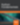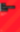# Java Language Basics Article

Java

Share:

#### Free JavaScript Book!Write powerful, clean and maintainable JavaScript.

RRP \$11.95

##### Operators

As I said above, any program that does anything useful will likely have to perform some kind of calculation, or manipulate information in some way or another. So far we’ve seen how to define pieces of information and store them in variables in preparation for that work to be done. In this section, I’ll introduce some of Java’s operators. Using operators you can take simple values (like numbers and strings of text) and perform operations on them (like addition, subtraction, or concatenation).

Let me show you a few simple examples so you know what I mean:

``````int a, b, c; // Three integer variables
a = 10;      // Assigns value 10 to a
b = 10 + 1;  // Assigns value 11 to b (+ is addition)
c = b - a;   // Assigns value 1 to c (- is subtraction)
a = b * 3;   // Assigns value 33 to a (* is multiplication)
c = a / b;   // Assigns value 3 to c (/ is division)``````

As a side note, you may have noticed that I used another shortcut on the first line of the above block of code to create three integer variables (`a`, `b`, and `c`) in a single statement. That one line could be expanded to the following, and it would work exactly the same:

``````int a;
int b;
int c;``````

Getting back to these operators, notice how I used the symbols +, -, *, and / to perform addition, subtraction, multiplication, and division respectively. These symbols are arithmetic operators. Notice also that operators may be used on literal values (`10 + 1`), variables (`b â€“ a`), or both (`b * 3`).

Another arithmetic operator that is less commonly used is the modulus operator:

``c = b % 3;   // Assigns value 2 to c (% is modulus op.)``

The modulus operator gives the remainder of an integer division. In this example, the value stored in `b` (11) divided by three is 9 with a remainder of 2 — this remainder is the value calculated by the `%` operator.

You can perform multiple operations in a single statement, if you want to. Note that arithmetic operations obey the same rules of precedence as they do on a scientific calculator: multiplication and division first, followed by addition and subtraction. Of course, we can use parentheses to change this if we want:

``````a = 1 + 2 * 3;   // a = 7
b = (1 + 2) * 3; // b = 9``````

Recall that variables don’t have to contain numbers. They can also hold strings of text… and operations can be performed on strings as well:

``````String name = "Kevin";
System.out.println("Hi " + name + ", how are you today?");``````

The code above displays the message `Hi Kevin, how are you today?` Notice how the `+` operator, which is used to add numbers together, can also be used to stick strings of text together (in other words, concatenate strings). Used in this context, `+` is called the string concatenation operator. Notice also that we’ve used the result of the expression `"Hi " + name + ", how are you today?"` as the value to be printed out by the `println` command. Nothing says we must store the result of an arithmetic calculation or other operation in a variable; as the example above shows, if we only need the value in one place we can simply type the expression where we need it.

Another family of operators is called the comparison operators. As their name suggests, these operators are used to compare different values together. While the arithmetic operators produce results that are numbers (usually `int` or `double`, depending on the data types that went into the operation) and the string concatenation operator produces `String`‘s, the comparison operators produce boolean values (i.e. either true or false). As before, some examples should illustrate this effectively:

``````// From above: a = 7, b = 9, and c = 2

boolean d, e, f; // Three boolean variables
d = (a == 7);    // d = true (== tests equality)
e = (a == b);    // e = false (a doesn't equal b)
f = (a != b);    // f = true (!= tests inequality)
d = (a > 0);     // d = true (a is greater than 0)
e = (b < 6);     // e = false (b is not less than 6)
f = (c >= 2);    // f = true (c is greater than or equal to 2)
d = (c <= 1);    // d = false (c is not less than or equal to 1)``````

As shown here, the comparison operators are `==`, `!=`, `>`, `<`, `>=`, and `<=`. Most of these operators only really make sense when applied to numbers (or variables containing numbers). One might expect, however, that `==` (test for equality) and `!=` (test for inequality) would work on `String` values, when in fact they don’t. While you won’t get an error if you try to compare strings using these operators, you’ll quickly discover that `==` will always turn out false (whether the string are the same or not), while `!=` will always give a true result. The reason for this, however, will have to wait for our next article, where I’ll explain objects and classes in all their glory.

You might be wondering about the practical uses of these comparison operators. In fact, they are essential when it comes to making your program do different things under different conditions. To accomplish such a feat, however, you’ll need one more tool in your belt: control structures.

Go to page: 1 | 2 | 3 | 4 | 5 | 6 | 7### Kevin Yank

Kevin Yank is an accomplished web developer, speaker, trainer and author of Build Your Own Database Driven Website Using PHP & MySQL and Co-Author of Simply JavaScript and Everything You Know About CSS is Wrong! Kevin loves to share his wealth of knowledge and it didn't stop at books, he's also the course instructor to 3 online courses in web development. Currently Kevin is the Director of Front End Engineering at Culture Amp.

#### New books out now!Learn valuable skills with a practical introduction to Python programming!Give yourself more options and write higher quality CSS with CSS Optimization Basics.